## Python math.isclose

##### Aniekan

In this comprehensive Python tutorial, we’ll explore the intricacies of comparing float values, shedding light on why relying on the ‘==’ operator for such comparisons can often yield unexpected outcomes. We’ll then introduce a robust solution: Python’s `math.isclose` function.

When delving into the realm of scientific computations in Python, one frequently encounters float values. However, comparing these values can be a daunting task due to the idiosyncrasies of how computers represent them.

Prepare to enhance your Python programming skills by mastering the art of precise float comparison. Let’s embark on this journey together, with ‘Python math.isclose’ as our guiding beacon.

## The problem with using `==` for `float` comparison

Internally, Python represents a `float` value using a finite binary representation. If we run the following code to print `0.1`,

``````print(0.1)
``````

We get the output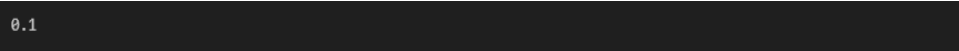However, if we want to see more of the fractional part, we can display it with 30 decimal places of precision.

``````print(f"{float(0.1):.30f}")
``````

we will get more details about the fractional part.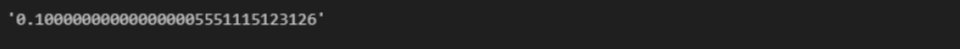Now we can see that internally, `0.1` is not represented as an exact value because it is represented internally using binary. Binary can only give an exact representation if the fractional number is a power of two or a sum of powers of two. For example:

``````# print 0.5 formatted as a string with 30 digits
# after the decimal place
print(f"{float(0.5):.30f}")

# evaluate 0.5+0.25 and print the result formatted
# as a string with 30 digits after the decimal place
print(f"{float(0.5+0.25):.30f}")

# evaluate 0.5+0.125 and print the result formatted
# as a string with 30 digits after the decimal place
print(f"{float(0.5+0.125):.30f}")
``````

The output is as follows: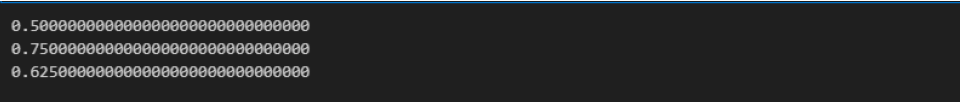From the output, we see that in this case, the representation is exact. However, Python can only represent `float` values like `0.1` approximately.

This raises a problem when trying to carry out comparisons using the `==` operator. The following code snippet illustrates the issue.

``````expr = 0.5 + 0.125
num = 0.625

print(f"0.5 + 0.125 (to 30dp) gives {expr:.30f}")
print(f"0.625 (to 30 dp) gives {num:.30f}")
print(f"expr==num evaluates to {expr==num}")
``````

The output we get is: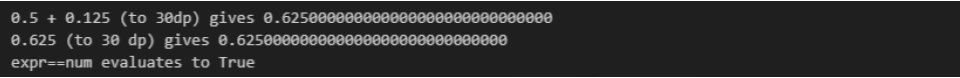From our output, we can see that the internal representation of `0.625` and the sum `0.5 + 0.125` are the same. So, comparison returns `True`.

The situation changes when we work with `float` values that are approximately represented internally, as the next code snippet shows.

``````expr = 0.1 + 0.2
num = 0.3

print(f"0.1 + 0.2 (to 30dp) gives {expr:.30f}")
print(f"0.3 (to 30 dp) gives {num:.30f}")
print(f"expr==num evaluates to {expr==num}")
``````

The output is as follows: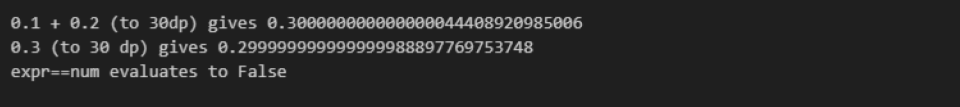The comparison returns `False` since the sum stored in `expr` is represented differently from the value stored in `num`, even if we intended to hold the same value of `0.3` in both variables.

The approximate representation of `float` values makes comparing them using the `==` operator unpredictable. Serious bugs could result if a situation similar to the above scenario occurred in our code. However, Python provides an alternative way that allows us to compare `float` values without running into this kind of bug.

## The solution: Python  `math.isclose` function

Python version 3.5 introduced the `math.isclose` function to address this challenge that arises when `float` values are compared. Instead of checking if the two numbers compared are the same, `math.isclose` checks if they are close to each other within a specified tolerance value.

The `math.isclose` Python function is called like so:

``math.isclose(a, b, rel_tol, abs_tol)``

Each parameter is explained below:

• `a` : The first value to compare for closeness (Required)
• `b` : The second value to compare for closeness (Required)
• `rel_tol` : The relative tolerance. The maximum allowed difference between `a` and `b` , relative to the bigger value compared. It has a default value of `1e-09` (Optional)
• `abs_tol` : The absolute tolerance. The maximum allowed difference between `a` and `b` . It has a default value of `0.0`

The function carries out the following calculation when doing comparisons:

``````abs_a, abs_b = abs(a), abs(b)
abs_tol_rel = rel_tol * max(abs_a, abs_b)

# The comparison...
abs(a - b) <= max(abs_tol_rel, abs_tol)
``````

If `a` and `b` are close, the comparison returns `True`.

## Examples of using Python `math.isclose` for `float` comparison

In the previous sections, we discussed the challenges of comparing `float` values using the equality operator, and how we could make use of an alternative, `math.isclose`, to check if two `float` numbers are close enough within a specific tolerance value. In this section, we will dive into examples of making use of `math.isclose`.

### Python Comparing two `float` values with default tolerances

The `math.isclose` function is defined with a default  `rel_tol`value of `1e-09` and a default `abs_tol` value of `0.0`. The next example will make use of these default tolerance settings to compare two `float` values.

``````from math import isclose

a = 0.1 + 0.1 + 0.1
b = 0.3

print(isclose(a, b))  # Output: True
``````

In the example, we used `math.isclose` to check if the sum `0.1 + 0.1 + 0.1` and the `float` literal `0.3` are close based on the default settings. The code prints out `True` which means that their internal representations are as close as allowed by the default tolerance values.

On the other hand, we will get a return value of `False` if we use `isclose`with default tolerance values to check if `1e-10` and `1e-11` are close.

``````result = isclose(1e-10, 1e-11)

print(result)  # Output: False
``````

From the tolerance formula discussed in the section that defines `math.isclose`, the absolute difference between `1e-10` and `1e-11` should be smaller than `max(rel_tol * max(1e-10, 1e-11), abs_tol)`. The absolute difference between `1e-10` and `1e-11` (a value of `9e-11`) is not smaller than the value returned by `max(rel_tol * max(1e-10, 1e-11), abs_tol)` (which is `1.0000000000000001e-19`). Therefore, `isclose` returns `False` for the comparison between `1e-10` and `1e-11`using the default tolerance values. Thus, the current tolerance values are not sufficient to consider `1e-10` and `1e-11` close.

### Python Comparing two `float` values with custom tolerance values

The default tolerance values work for many situations. However, different values can be passed to `math.isclose` to suit specific requirements. Let’s explore examples that pass different values for `rel_tol` and `abs_tol`.

Hers an example that compares two values `1000.0` and `1001.0` using `rel_tol` value `1e-5`. Running the code snippet will show how the comparison plays out.

``````from math import isclose

result = isclose(1000.0, 1001.0, rel_tol=1e-5)

print(result)  # Output: False
``````

Here, `math.isclose` returns `False` because the absolute difference between `1000.0` and `1001.0` relative to the magnitude of the bigger number `1001.0`does not fall within the specified relative tolerance.

This next example finds out if the sum `0.1 + 0.2` is within `1e-10` of `0.3`.

``````from math import isclose

result = isclose(0.1+0.2, 0.3, abs_tol=1e-10)

print(result)  # Output: True
``````

`math.isclose` returns `True` since the absolute difference between the internal representation of the result of `0.1 + 0.2` and the internal representation of `0.3`  is smaller than`1e-10` (the `abs_tol`).

In conclusion `math.isclose` offers a solution arising when trying to compare `float` values. Instead of directly checking for equality

, the function checks if the absolute difference of the compared values falls under a tolerance value, effectively overcoming rounding errors and precision issues. Understanding the use of `math.isclose` is crucial if we want to avoid bugs when carrying out float comparison. in our code. If you liked this tutorial, please consider checking out other tutorials like this on our website that can help you in your coding journey.